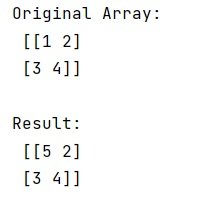# numpy.mat() Method with Example

Learn about the numpy.mat() method with its usage, syntax, and example.
Submitted by Pranit Sharma, on March 21, 2023

## numpy.mat() Method

The numpy.mat() method interprets the given input as a matrix. It is equivalent to numpy.matrix(). It does not make a copy of the data if the data is already a matrix or a ndarray.

## Syntax

```numpy.mat(data, dtype=None)
```

## Parameter(s)

• data: Represents the input data of array_like type.
• dtype: Represents the type of the output matrix.

## Return Value

It returns data interpreted as a matrix.

## Example of numpy.mat() method in Python

```# Import numpy
import numpy as np

# Creating a numpy array
arr = np.array([[1, 2], [3, 4]])

# Display original array
print("Original Array:\n",arr,"\n")

# Creating a matrix using mat
res = np.mat(arr)

# Display result
arr[0,0] = 5
print("Result:\n",res)
```

### Output